﻿ 近水面低航速下潜航器深度自抗扰控制
 舰船科学技术2020, Vol. 42Issue (1): 28-31PDF

ADRC based control of a low-speed underwater flight vehicle near surface
ZHAO Shuo, FENG Zheng-ping, ZHENG Tian-hai, PAN Wan-jun
School of Marine and Architectural Engineering, Shanghai Jiaotong University, Shanghai 200240, China
Abstract: The performance of depth control for an underwater flight vehicle (UFV) is important. Due to the considerable wave-suction force, depth control of an underwater flight vehicle moving near surface and disturbed by wave is extremely hard. Meanwhile, the lift force generated by the bow and stern planes will decrease significantly due to low efficiency under low speeds. A depth controller of UFV based on active disturbance rejection controller (ADRC) is designed in this paper. The extended state observer observes and compensates the disturbance of wave on line. Both the results of simulations and tank tests of depth control of an underwater flight vehicle verify the advantages of the active disturbance rejection controller over conventional PID controller.
Key words: underwater flight vehicle     active disturbance rejection controller     depth control     extended state observer
0 引　言

PID控制方法凭借着不依赖模型参数、适应范围广、简单易用的优势在众多领域得到了广泛的应用。其控制思想是根据控制目标与实际行为之间的误差来确定消除此误差的控制量。然而，PID方法也存在着诸多缺点。首先，该方法是根据已经存在的偏差来计算纠正偏差的控制量，因而产生滞后。其次，PID中微分项的获取在工程中并不容易，处理不当会产生噪声放大的不良效果。同时，为保证消除稳态误差而存在的积分项可能会导致积分饱和等现象。最后，PID三项线性加权的方式未必能组合出最佳的控制量。面对PID方法存在的缺点，国内外已经有多种控制方法被提出，包括模糊PID与滑模等控制方法

1 问题的提出

 \left\{ {\begin{aligned} & {\dot z = - u \cdot \sin \theta + w \cdot {\rm{cos}}\theta }{\text{，}}\\ & {\left( {m - {Z_{\dot w}}} \right)\dot w - {Z_w}w - {Z_{\left| w \right|w}}\left| w \right|w - {Z_{\dot q}}\dot q - }\\ & \quad \quad{{Z_q}q = {\delta _s}{Z_{{\delta _s}}} + {\delta _b}{Z_{{\delta _b}}} + {Z_0}}{\text{。}} \end{aligned}} \right. (1)

2 自抗扰控制器设计

1）跟踪微分器可以提取微分信号，并且相对于传统差分的方法具有噪声放大效果不明显的优势，同时还可以安排过渡过程以避免由于初始偏差过大引起的超调问题。

 $\left\{ {\begin{split} &{{v_1}\left( {k \!+\! 1} \right) \!=\! {v_1}\left( k \right) \!+\! h\cdot{v_2}\left( k \right)}{\text{，}}\\ &{{v_2}\left( {k \!+\! 1} \right) \!=\! {v_2}\left( k \right) \!+\! h\cdot {\rm{fhan}}\left( {{v_1}\left( k \right) \!-\! v\left( k \right),{v_2}\left( k \right),r,h} \right)}{\text{。}} \end{split}} \right.$ (2)

2）扩张状态观测器能够观测控制目标的值及其微分信号，并且将除控制输入量以外的合力作为总扰动（包括模型误差与环境扰动力）观测出以便给予补偿。

 \left\{ {\begin{aligned} & {e = {z_1} - y}{\text{，}}\\ & {\mathop {{z_1}}\limits^ \cdot = {z_2} - {\beta _{01}}e}{\text{，}}\\ & {\mathop {{z_2}}\limits^ \cdot = {z_3} - {\beta _{02}}{f_1} + bu\left( t \right)}{\text{，}}\\ & {{z_3} = - {\beta _{03}}fal\left( {e,\alpha ,\delta } \right)}{\text{。}} \end{aligned}} \right. (3)

3）非线性加权能够将控制目标的偏差及其微分信号进行高效的组合以产生合适的控制输入量。

 $\left\{ {\begin{array}{*{20}{l}} {{e_1} = {v_1}\left( k \right) - {z_1}\left( k \right)}{\text{，}}\\ {{e_2} = {v_2}\left( k \right) - {z_2}\left( k \right)}{\text{，}}\\ {{u_0}\left( k \right) = {\beta _{01}}fal\left( {{e_1},{\alpha _1},\delta } \right) + {\beta _{02}}fal\left( {{e_1},{\alpha _2},\delta } \right)}{\text{，}}\\ {u\left( k \right) = {u_0} - \dfrac{{{z_3}\left( k \right)}}{b}}{\text{。}} \end{array}} \right.$ (4)

 $\left\{ {\begin{array}{*{20}{l}} {{v_1}\left( {k + 1} \right) = {v_1}\left( k \right) + h\cdot{v_2}\left( k \right)}{\text{，}}\\ {{v_2}\left( {k + 1} \right) = {v_2}\left( k \right)} +\\ \qquad\qquad\;\;\;{ h\cdot fhan\left( {{v_1}\left( k \right) - v\left( k \right),{v_2}\left( k \right),r,{h_0}} \right)}{\text{。}} \end{array}} \right.$ (5)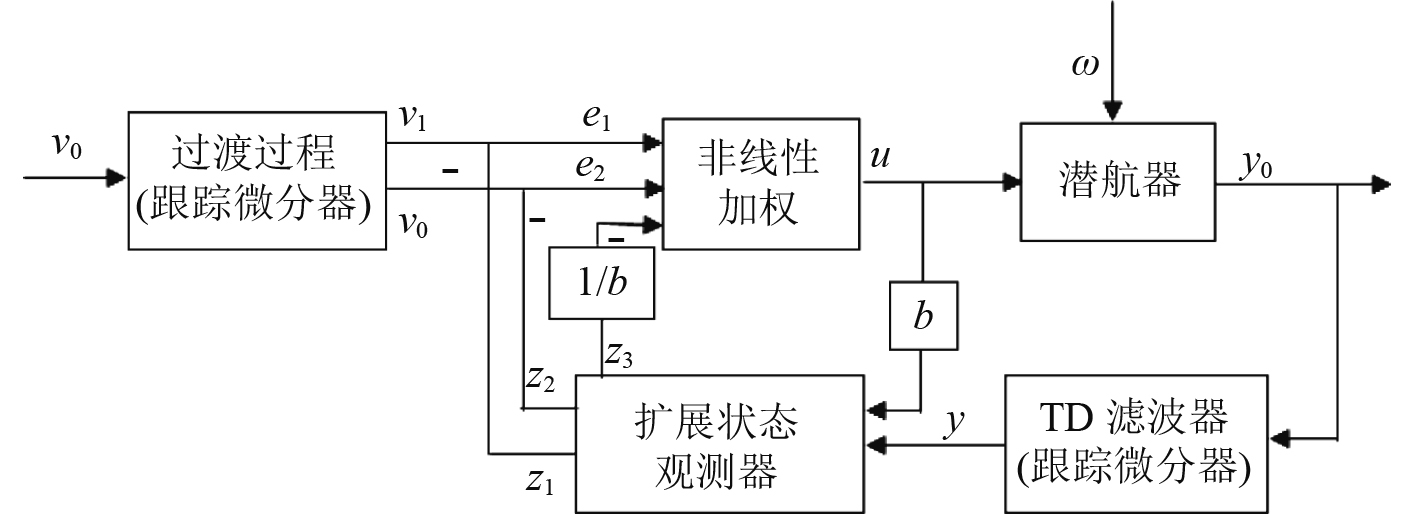图 1 自抗扰控制器结构图 Fig. 1 Structure of ADRC
3 仿　真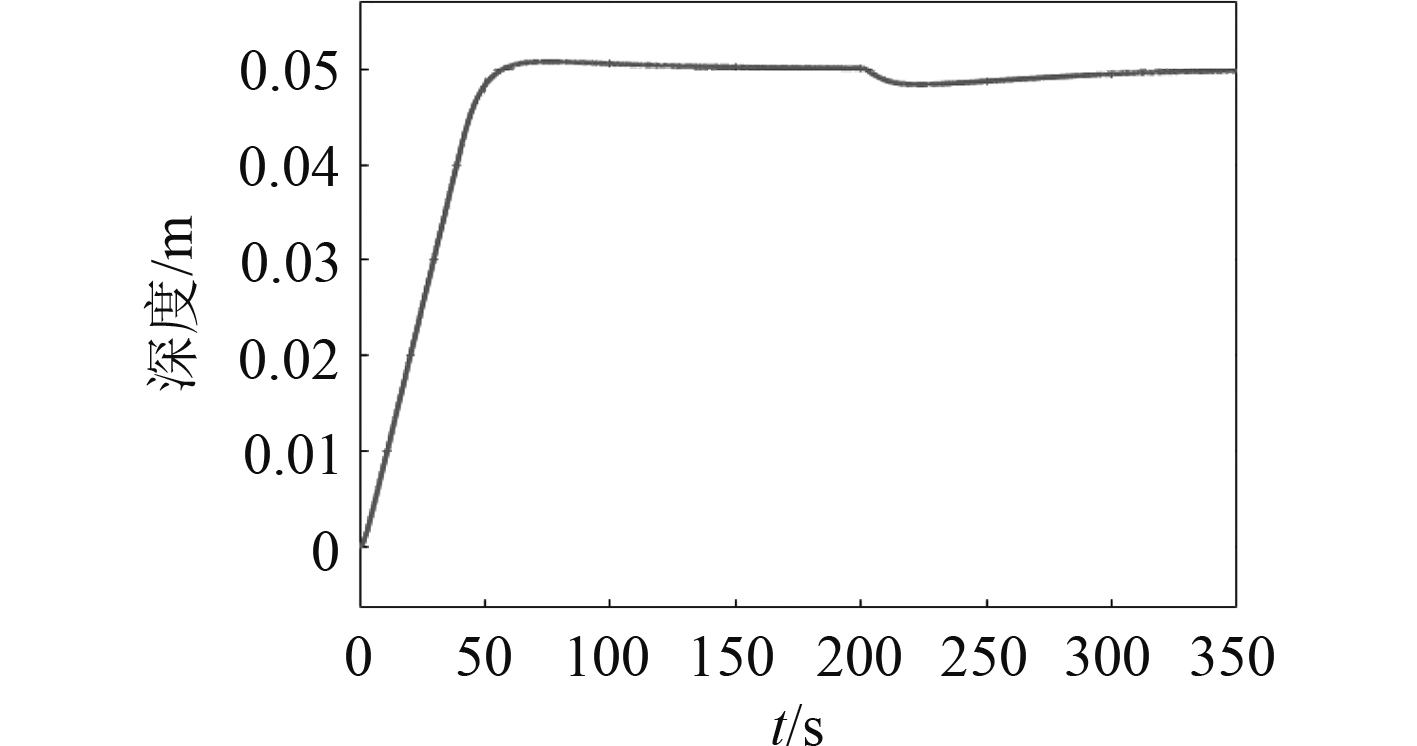图 2 PID控制器深度控制效果 Fig. 2 Depth (PID)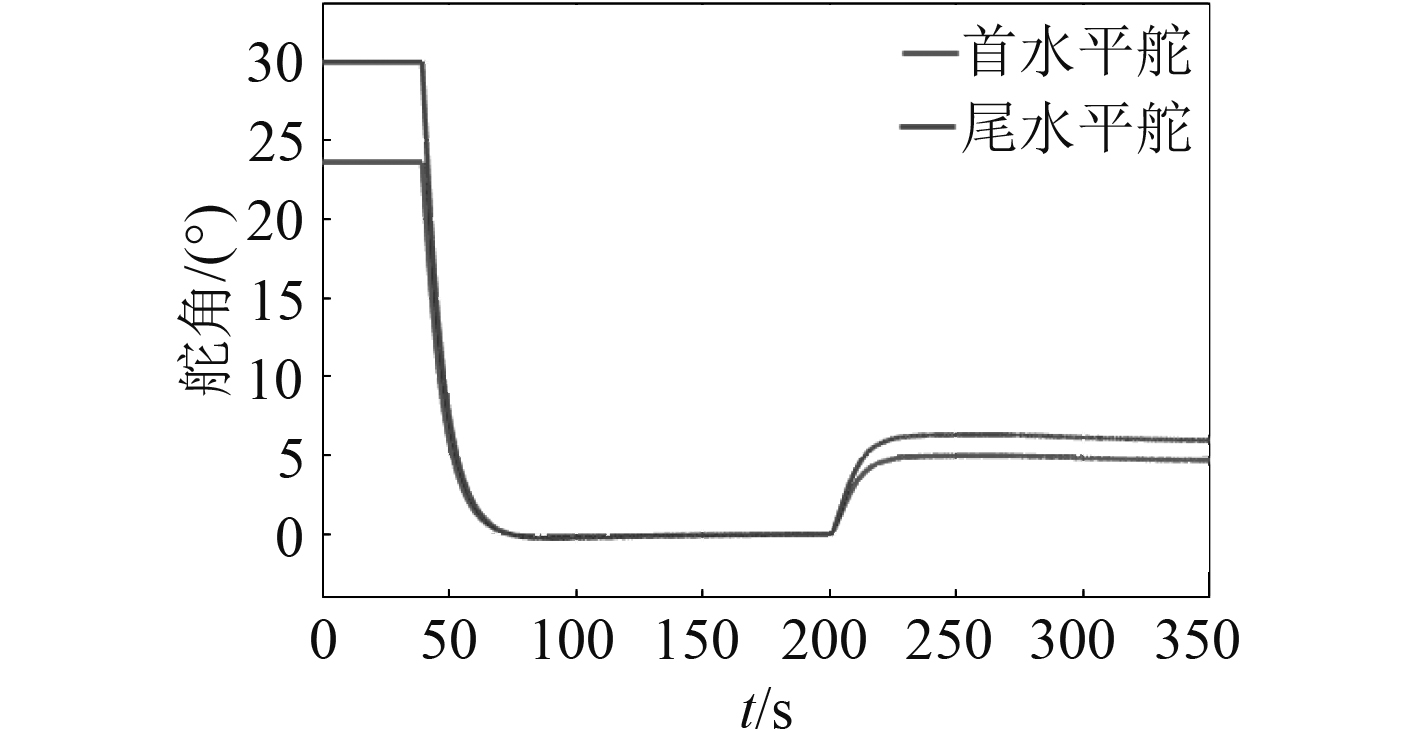图 3 PID控制器水平舵舵角变化 Fig. 3 Angle of planes (PID)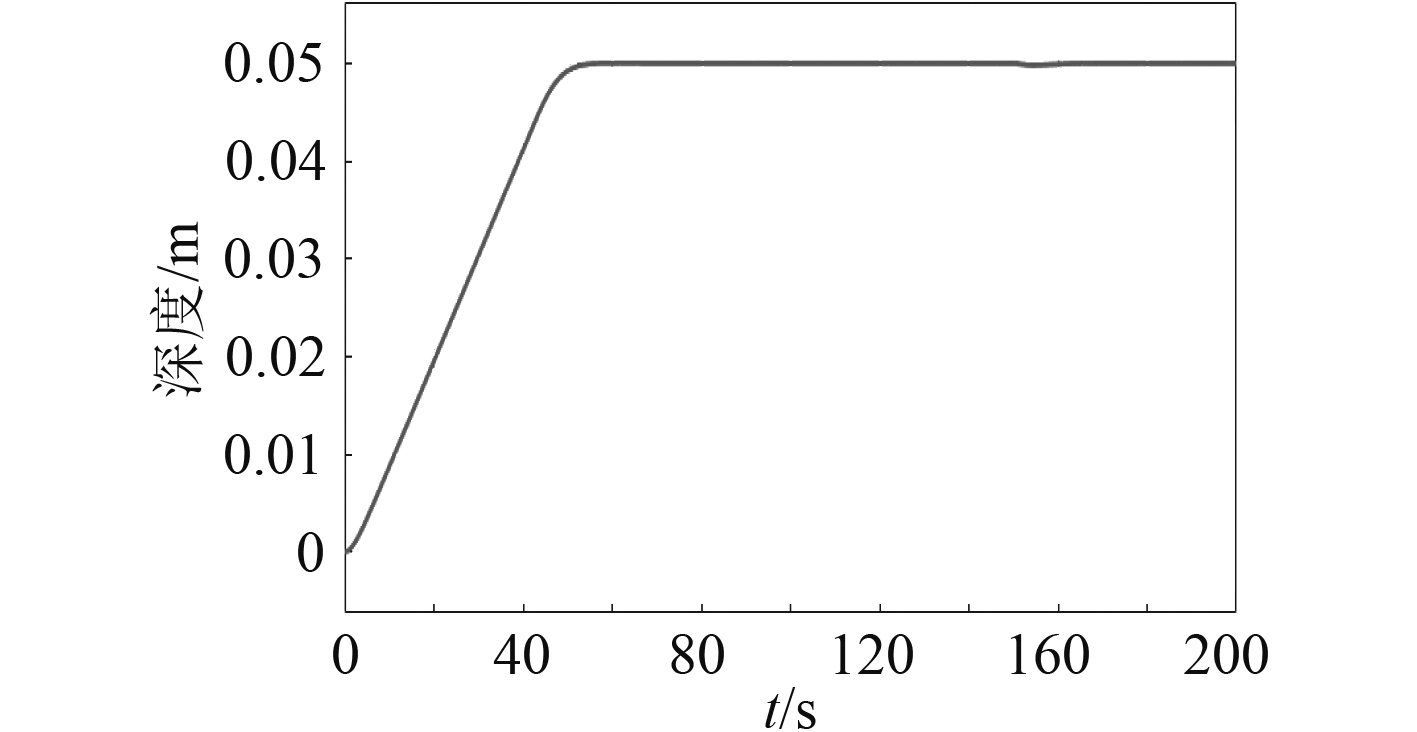图 4 深度控制效果 Fig. 4 Deprh (ADRC)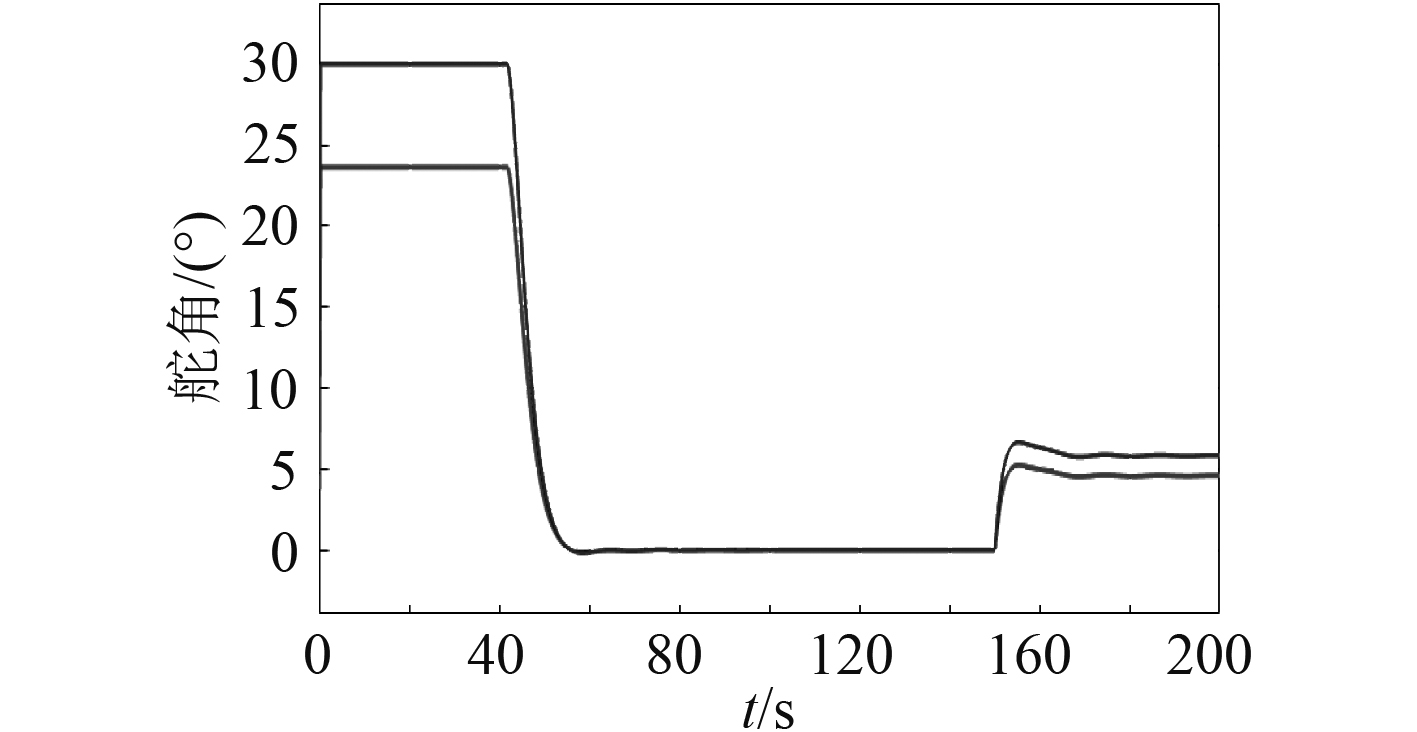图 5 ADRC控制器水平舵舵角变化 Fig. 5 Angle of planes (ADRC)

4 实　验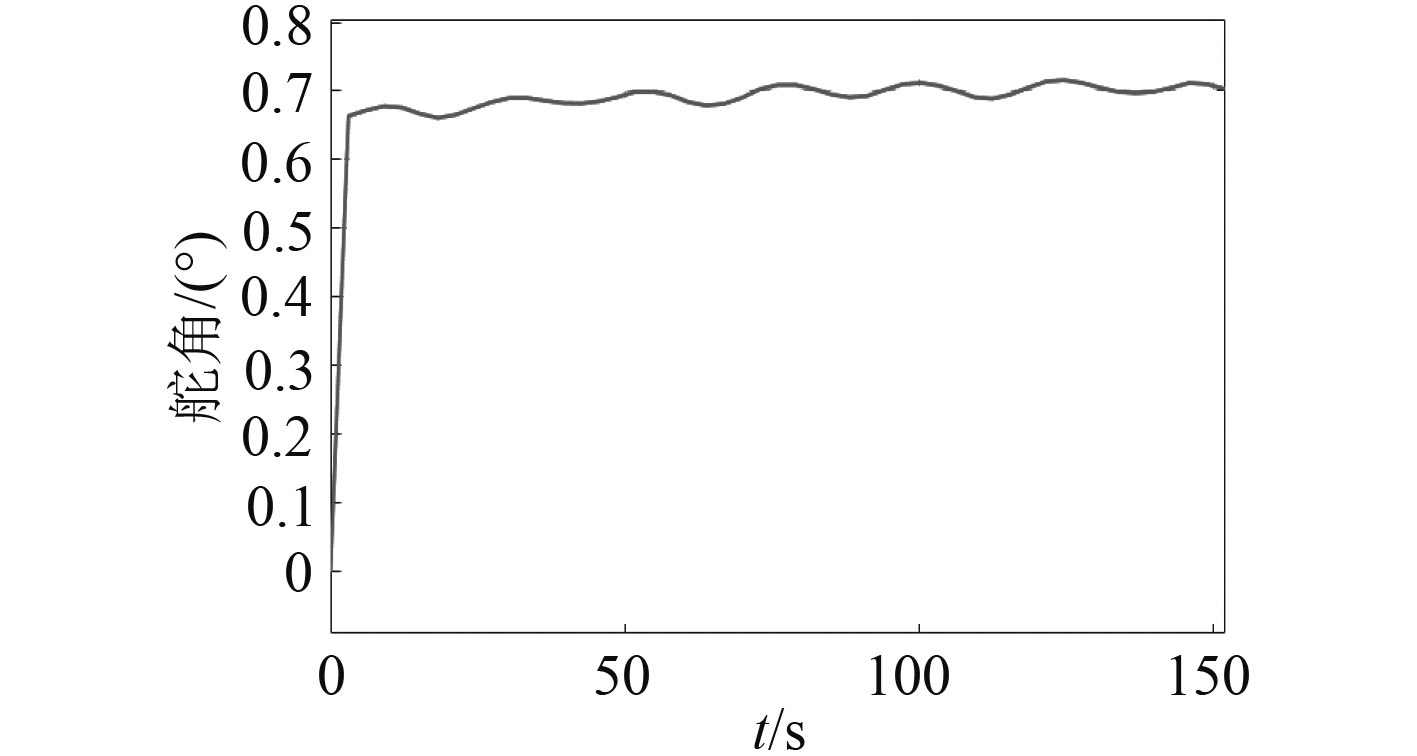图 6 深度控制实验效果 Fig. 6 Depth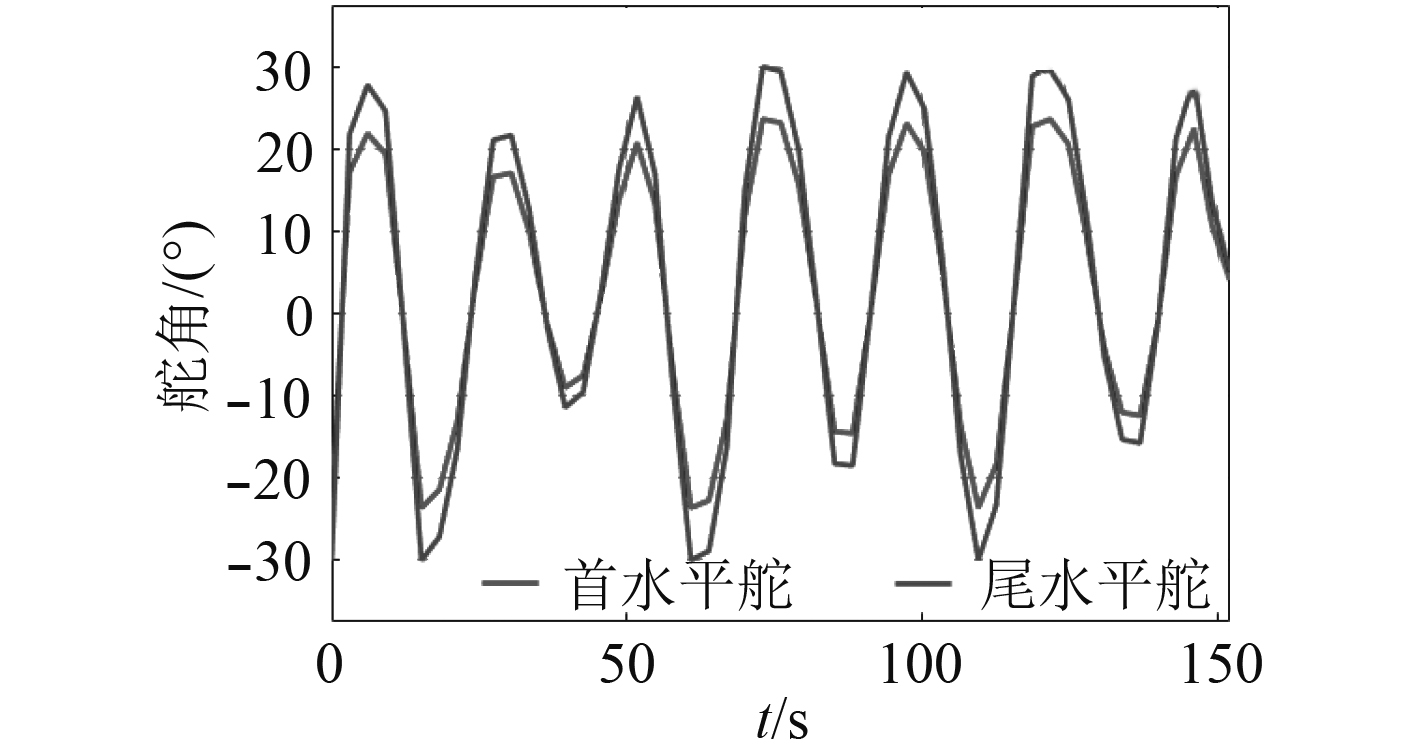图 7 水平舵舵角变化实验结果 Fig. 7 Angle of planes

5 结　语

  ZHANG J, LIAN L, TONG G. Robust control and simulation of near-surface submarine[J]. Ocean Engineering, 2006, 24(4): 32-31.  LICÉAGA-CASTRO E, UGALDE-LOO C E, NAVARRO-LÓPEZ E M. Efficient multivariable submarine depth-control system design[J]. Ocean Engineering, 2008, 35(17): 1747-1758.  GAO Fu-dong, PAN Cun-yun, HAN Yan-yan, et al. Nonlinear trajectory tracking control of a new autonomous underwater vehicle in complex sea conditions[J]. Journal of Central South University, 2012, 19(7): 1859-1868. DOI:10.1007/s11771-012-1220-1  FONT R, GARCÍA-PELÁEZ J. On a submarine hovering system based on blowing and venting of ballast tanks[J]. Ocean Engineering, 2013, 72(7): 441-447.  林龙信, 沈林成, 张代兵. 仿生波动鳍的试验研究[J]. 哈尔滨工程大学学报, 2008, 29(5): 489-492. DOI:10.3969/j.issn.1006-7043.2008.05.011  JIN H, PAN L, WANG L. Adaptive variable structure control with neuron for low speed stabilizing of submarine near surface[J]. Control & Decision, 2010, 25(4): 562-566+571.  LICEAGA-CASTRO E, VAN d M G M. Submarine H ∞, depth control under wave disturbances[J]. Control Systems Technology IEEE Transactions on, 1995, 3(3): 338-346. DOI:10.1109/87.406981  HAN J. From PID to Active Disturbance Rejection Control[J]. IEEE Transactions on Industrial Electronics, 2009, 56(3): 900-906. DOI:10.1109/TIE.2008.2011621  LIN X, HU D, DUAN Z. The Research on Fuzzy-PID Control of the Submarine Course[C]// International Symposium on Computational Intelligence & Design. IEEE, 2013: 281-284.  ASHRAFIUON H, MUSKE K R, MCNINCH L C, et al. Sliding-Mode Tracking Control of Surface Vessels[J]. IEEE Transactions on Industrial Electronics, 2008, 55(11): 4004-4012. DOI:10.1109/TIE.2008.2005933  XUE W, BAI W, YANG S, et al. ADRC with adaptive extended state observer and its application to air-fuel ratio control in gasoline engines[J]. IEEE Transactions on Industrial Electronics, 2015, 62(9): 5847-5857. DOI:10.1109/TIE.2015.2435004  CUI R, CHEN L, YANG C, et al. Extended state observer-based integral sliding mode control for an underwater robot with unknown disturbances and uncertain nonlinearities[J]. IEEE Transactions on Industrial Electronics, 2017, 64(8): 6785-6795. DOI:10.1109/TIE.2017.2694410  DUAN W, WANG L, CHEN J. Calculation of vertical second-order drift loads on a submarine floating near the free water surface based on Taylor expansion boundary element method[J]. Journal of Harbin Engineering University, 2017, 38(1): 8-12.  T I. FROSSEN, Guidance and control of ocean vehicles. 1994.  L. ZHANG, Y B. LI. Fluid Mechanics, 220-221, 2006  L. WU, J. HAN. TD Filter and its applications[J]. Computing Technology and Automation, 2003, 22(s1): 61-63.